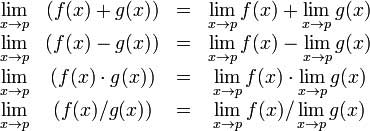# MATLAB - Calculus

MATLAB provides various ways for solving problems of differential and integral calculus, solving differential equations of any degree and calculation of limits. Best of all, you can easily plot the graphs of complex functions and check maxima, minima and other stationery points on a graph by solving the original function, as well as its derivative.

This chapter will deal with problems of calculus. In this chapter, we will discuss pre-calculus concepts i.e., calculating limits of functions and verifying the properties of limits.

In the next chapter Differential, we will compute derivative of an expression and find the local maxima and minima on a graph. We will also discuss solving differential equations.

Finally, in the Integration chapter, we will discuss integral calculus.

## Calculating Limits

MATLAB provides the limit function for calculating limits. In its most basic form, the limit function takes expression as an argument and finds the limit of the expression as the independent variable goes to zero.

For example, let us calculate the limit of a function f(x) = (x3 + 5)/(x4 + 7), as x tends to zero.

```syms x
limit((x^3 + 5)/(x^4 + 7))
```

MATLAB will execute the above statement and return the following result −

```ans =
5/7
```

The limit function falls in the realm of symbolic computing; you need to use the syms function to tell MATLAB which symbolic variables you are using. You can also compute limit of a function, as the variable tends to some number other than zero. To calculate lim x->a(f(x)), we use the limit command with arguments. The first being the expression and the second is the number, that x approaches, here it is a.

For example, let us calculate limit of a function f(x) = (x-3)/(x-1), as x tends to 1.

```limit((x - 3)/(x-1),1)
```

MATLAB will execute the above statement and return the following result −

```ans =
NaN
```

Let's take another example,

```limit(x^2 + 5, 3)
```

MATLAB will execute the above statement and return the following result −

```ans =
14
```

## Calculating Limits using Octave

Following is Octave version of the above example using symbolic package, try to execute and compare the result −

```pkg load symbolic
symbols

x = sym("x");
subs((x^3+5)/(x^4+7),x,0)
```

Octave will execute the above statement and return the following result −

```ans =
0.7142857142857142857
```

## Verification of Basic Properties of Limits

Algebraic Limit Theorem provides some basic properties of limits. These are as follows −Let us consider two functions −

• f(x) = (3x + 5)/(x - 3)
• g(x) = x2 + 1.

Let us calculate the limits of the functions as x tends to 5, of both functions and verify the basic properties of limits using these two functions and MATLAB.

### Example

Create a script file and type the following code into it −

```syms x
f = (3*x + 5)/(x-3);
g = x^2 + 1;
l1 = limit(f, 4)
l2 = limit (g, 4)
lAdd = limit(f + g, 4)
lSub = limit(f - g, 4)
lMult = limit(f*g, 4)
lDiv = limit (f/g, 4)
```

When you run the file, it displays −

```l1 =
17

l2 =
17

34

lSub =
0

lMult =
289

lDiv =
1
```

## Verification of Basic Properties of Limits using Octave

Following is Octave version of the above example using symbolic package, try to execute and compare the result −

```pkg load symbolic
symbols

x = sym("x");
f = (3*x + 5)/(x-3);
g = x^2 + 1;

l1 = subs(f, x, 4)
l2 = subs (g, x, 4)
lAdd = subs (f+g, x, 4)
lSub = subs (f-g, x, 4)
lMult = subs (f*g, x, 4)
lDiv = subs (f/g, x, 4)
```

Octave will execute the above statement and return the following result −

```l1 =
17.0
l2 =
17.0
34.0
lSub =
0.0
lMult =
289.0
lDiv =
1.0
```

## Left and Right Sided Limits

When a function has a discontinuity for some particular value of the variable, the limit does not exist at that point. In other words, limits of a function f(x) has discontinuity at x = a, when the value of limit, as x approaches x from left side, does not equal the value of the limit as x approaches from right side.

This leads to the concept of left-handed and right-handed limits. A left-handed limit is defined as the limit as x -> a, from the left, i.e., x approaches a, for values of x < a. A right-handed limit is defined as the limit as x -> a, from the right, i.e., x approaches a, for values of x > a. When the left-handed limit and right-handed limit are not equal, the limit does not exist.

Let us consider a function −

f(x) = (x - 3)/|x - 3|

We will show that limx->3 f(x) does not exist. MATLAB helps us to establish this fact in two ways −

• By plotting the graph of the function and showing the discontinuity.
• By computing the limits and showing that both are different.

The left-handed and right-handed limits are computed by passing the character strings 'left' and 'right' to the limit command as the last argument.

### Example

Create a script file and type the following code into it −

```f = (x - 3)/abs(x-3);
ezplot(f,[-1,5])
l = limit(f,x,3,'left')
r = limit(f,x,3,'right')
```

When you run the file, MATLAB draws the following plotAfter this following output is displayed −

```l =
-1

r =
1
```